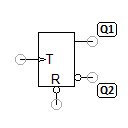# T flip-flop with inverted RESET inputThis T flip-flop is a T flip-flop with an additional input R (reset), via which the output Q1 can be set directly to 0. The input R is inverted, i.e. R=0 sets Q1 directly to 0.

Truth table

S T Q1 Q2
0 x 0 1
1 q2 q1
1 x q1 q2

x = any (0 or 1) ↑ = positive edge from 0 to 1 q = state of output Q before the edge